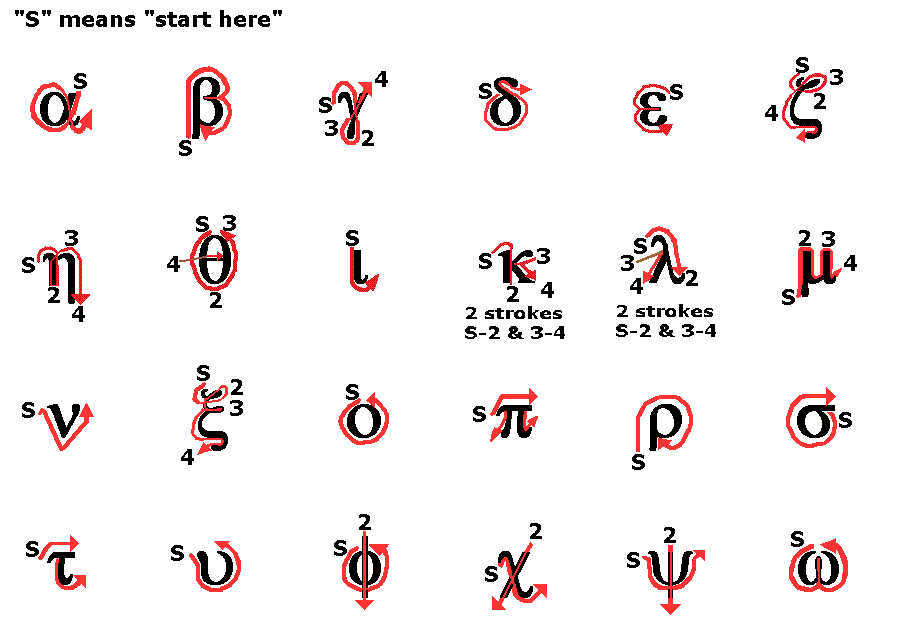# 关于希腊字母的那些事

在很多公式中都会出现希腊字母，比如最常见的圆周率 $\pi$ 就是其中之一。但是，学到后面，用的希腊字母就越用越多，让我很头晕。所以就想仔细总结一下各个希腊字母的知识。

# 希腊字母的历史

希腊字母大约是在公元前 1000 年左右形成的，它是由 Phoenician Alphabet 演化而来，之后又从它演化出了现在欧洲各语言的字母表。# 希腊字母的写法

希腊字母共有 24 个，和英文字母一样存在大小写，具体如下：图中，最大的是大写希腊字母，旁边的是小写希腊字母，同时还标注了在英文中的名称和常见读音。比如，最常见的 $\pi$，其大写形式为 $\Pi$，英文名称为 Pi。顺便说一句，在 LaTeX 中，可以用 \pi（小写） 和 \Pi（大写）的形式来输入 $\pi$，类比可以输入其他希腊字母。

除了上面列出的形式外，还有一些异体写法，包括如下 7 个：

$\epsilon$ $\varepsilon$ \varepsilon
$\kappa$ $\varkappa$ \varkappa
$\theta$ $\vartheta$ \vartheta
$\pi$ $\varpi$ \varpi
$\rho$ $\varrho$ \varrho
$\sigma$ $\varsigma$ \varsigma
$\phi$ $\varphi$ \varphi

至于这些在纸上怎么写，可以参考下图或Greek Handwriting。这里面最难写的就是 $\xi$ 和 $\zeta$，我自己的写法是 $\xi$ 写成 “ε”，收笔时向左下画一撇；$\zeta$ 则写成一个“7”，收笔时同样向左下画一撇。# 希腊字母的读音

我们上面已经给出了希腊字母的常见读音，平时照着那个英文读就行。但但但是，那个读音很多都是错的。比如说我们最常见的 $\pi$，几乎所有人都读成“派” [paɪ]，但正确的读音应该是“劈”[pi]，和英语中 pee（小便）的读音一样。详细的读音请看下面的视频：

当然，从沟通的角度，我觉得读成习惯的音即可。不知道怎么读也没事，最多就上课回答问题时有点尴尬。

# 希腊字母的意义

各个希腊字母在不同学科中有不同意义，这个是真的难记。如果有人想要总结的话，我找个时间翻翻书……